# Basic Combinations

Since the raising of a number n to a power p may be defined as multiplying n times itself p times, it follows that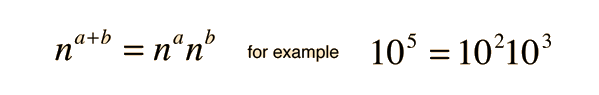The rule for raising a power to a power can also be deduced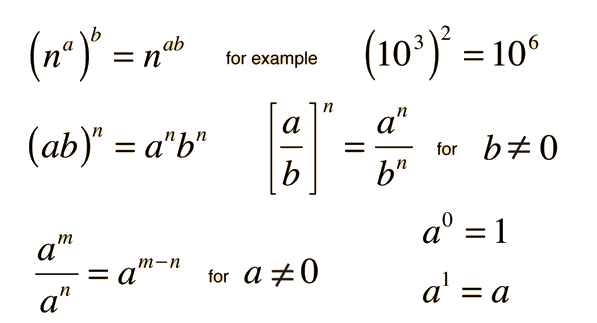Index

 HyperPhysics*****HyperMath *****Exponents R Nave
Go Back

# Powers of Ten

For very large or very small numbers, it convenient to use the "scientific notation", i.e., to express the numbers in terms of a power of 10. Standard prefixes are used to denote certain powers of 10:Index

 HyperPhysics*****HyperMath *****Exponents R Nave
Go Back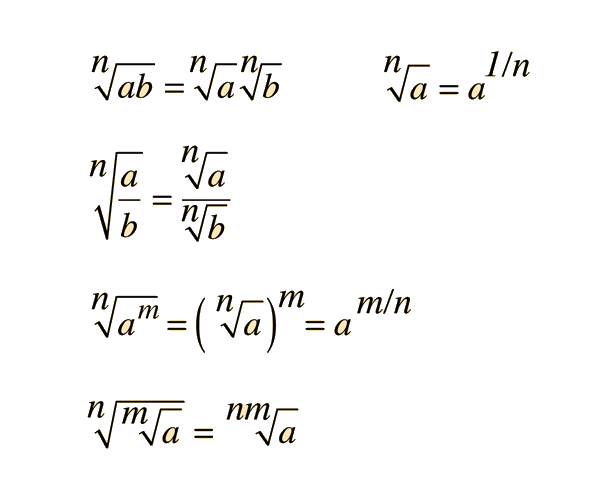Index

 HyperPhysics*****HyperMath *****Exponents R Nave
Go Back

# Euler Relationship

The trigonometric functions are related to a complex exponential by the Euler relationship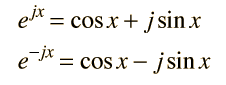From these relationships the trig functions can be expressed in terms of the complex exponential: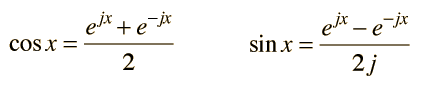Index

 HyperPhysics*****HyperMath *****Exponents R Nave
Go Back

# Hyperbolic Functions

The hyperbolic functions can be defined in terms of exponentials.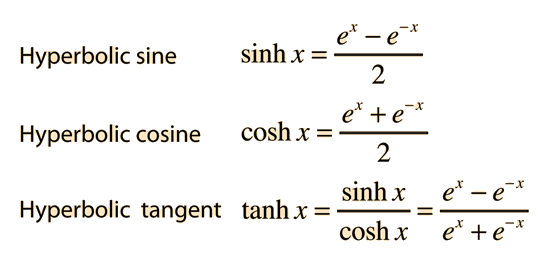There are closed-form expressions for the inverse hyperbolic functions:An application of the hyperbolic tangent is in calculating the speed of ocean waves. The hyperbolic cosine and its inverse are encountered in fluid friction in the case of quadratic drag.

Index

 HyperPhysics*****HyperMath *****Exponents R Nave
Go Back